### Jag Test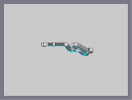Hover over the thumbnail for a full-size version.

Author RavenMac author:ravenmac n-art unrated 2008-07-04 2008-07-04 \$Jag Test#RavenMac#none#00000000000000000000000000000000000000000000000000000000000000000000000000000000000000000000000000000000000000000000000000000000000000000000000000000000000000000000000000000000000000000000000000000000000000000000000000000000000000000000000000000000000000000000000000000000000000000000000000000000000000000000000000000000000000000000000000000000000000000000000000000000000000000000000000000000000000000000000000000000000000000000000000000000000000000000000000000000000000000000000000000000000000000000000000000000000000000000000000000000000000000000000000000000000000000000000000000000000000000000000000000000000000000000000000000000000000000000000000000000000000000000000000000000000000000000000000000000000000000|8^510,325,1!1^511,324!8^511,325,1!1^512,324!8^512,325,1!1^513,324!8^513,325,1!1^514,324!8^514,325,1!1^515,324!1^516,325!8^505,324,1!1^506,323!8^506,324,1!1^507,323!8^507,324,1!1^508,323!8^508,324,1!1^509,323!8^509,324,1!1^510,323!8^510,324,1!1^511,323!8^511,324,1!1^512,323!8^512,324,1!1^513,323!8^513,324,1!1^514,323!8^514,324,1!1^515,323!8^515,324,1!1^516,323!8^516,324,1!1^517,323!8^517,324,1!1^518,323!1^519,324!8^503,323,1!1^504,322!8^504,323,1!1^505,322!8^505,323,1!1^506,322!8^506,323,1!1^507,322!8^507,323,1!1^508,322!8^508,323,1!1^509,322!8^509,323,1!1^510,322!8^510,323,1!1^511,322!8^511,323,1!1^512,322!8^512,323,1!1^513,322!8^513,323,1!1^514,322!8^514,323,1!1^515,322!8^515,323,1!1^516,322!8^516,323,1!1^517,322!8^517,323,1!1^518,322!8^518,323,1!1^519,322!8^519,323,1!1^520,322!1^521,323!8^501,322,1!1^502,321!8^502,322,1!1^503,321!8^503,322,1!1^504,321!8^504,322,1!1^505,321!8^505,322,1!1^506,321!8^506,322,1!1^507,321!8^507,322,1!1^508,321!8^508,322,1!1^509,321!8^509,322,1!1^510,321!8^510,322,1!1^511,321!8^511,322,1!1^512,321!8^512,322,1!1^513,321!8^513,322,1!1^514,321!8^514,322,1!1^515,321!8^515,322,1!1^516,321!8^516,322,1!1^517,321!8^517,322,1!1^518,321!8^518,322,1!1^519,321!8^519,322,1!1^520,321!1^521,322!8^499,321,1!1^500,320!8^500,321,1!1^501,320!8^501,321,1!1^502,320!8^502,321,1!1^503,320!8^503,321,1!1^504,320!8^504,321,1!1^505,320!8^505,321,1!1^506,320!8^506,321,1!1^507,320!8^507,321,1!1^508,320!8^508,321,1!1^509,320!8^509,321,1!1^510,320!8^510,321,1!1^511,320!8^511,321,1!1^512,320!8^512,321,1!1^513,320!8^513,321,1!1^514,320!8^514,321,1!1^515,320!8^515,321,1!1^516,320!8^516,321,1!1^517,320!8^517,321,1!1^518,320!8^518,321,1!1^519,320!8^519,321,1!1^520,320!8^520,321,1!1^521,320!1^522,321!8^497,320,1!1^498,319!8^498,320,1!1^499,319!8^499,320,1!1^500,319!8^500,320,1!1^501,319!8^501,320,1!1^502,319!8^502,320,1!1^503,319!1^504,320!8^512,320,1!1^513,319!8^513,320,1!1^514,319!8^514,320,1!1^515,319!8^515,320,1!1^516,319!8^516,320,1!1^517,319!8^517,320,1!1^518,319!8^518,320,1!1^519,319!8^519,320,1!1^520,319!8^520,320,1!1^521,319!1^522,320!8^496,319,1!1^497,318!8^497,319,1!1^498,318!8^498,319,1!1^499,318!8^499,319,1!1^500,318!1^501,319!8^513,319,1!1^514,318!8^514,319,1!1^515,318!8^515,319,1!1^516,318!8^516,319,1!1^517,318!8^517,319,1!1^518,318!8^518,319,1!1^519,318!8^519,319,1!1^520,318!8^520,319,1!1^521,318!8^521,319,1!1^522,318!1^523,319!8^494,318,1!1^495,317!8^495,318,1!1^496,317!8^496,318,1!1^497,317!8^497,318,1!1^498,317!1^499,318!8^514,318,1!1^515,317!8^515,318,1!1^516,317!8^516,318,1!1^517,317!8^517,318,1!1^518,317!8^518,318,1!1^519,317!8^519,318,1!1^520,317!8^520,318,1!1^521,317!8^521,318,1!1^522,317!1^523,318!8^493,317,1!1^494,316!8^494,317,1!1^495,316!8^495,317,1!1^496,316!1^497,317!8^515,317,1!1^516,316!8^516,317,1!1^517,316!8^517,317,1!1^518,316!8^518,317,1!1^519,316!8^519,317,1!1^520,316!8^520,317,1!1^521,316!8^521,317,1!1^522,316!1^523,317!8^491,316,1!1^492,315!8^492,316,1!1^493,315!8^493,316,1!1^494,315!8^494,316,1!1^495,315!1^496,316!8^515,316,1!1^516,315!8^516,316,1!1^517,315!8^517,316,1!1^518,315!8^518,316,1!1^519,315!8^519,316,1!1^520,315!8^520,316,1!1^521,315!8^521,316,1!1^522,315!1^523,316!8^490,315,1!1^491,314!8^491,315,1!1^492,314!8^492,315,1!1^493,314!8^493,315,1!1^494,314!1^495,315!8^515,315,1!1^516,314!8^516,315,1!1^517,314!8^517,315,1!1^518,314!8^518,315,1!1^519,314!8^519,315,1!1^520,314!8^520,315,1!1^521,314!1^523,315!8^489,314,1!1^490,313!8^490,314,1!1^491,313!8^491,314,1!1^492,313!1^493,314!8^515,314,1!1^516,313!8^516,314,1!1^517,313!8^517,314,1!1^518,313!8^518,314,1!1^519,313!8^519,314,1!1^520,313!8^520,314,1!1^521,313!1^523,314!8^488,313,1!1^489,312!8^489,313,1!1^490,312!8^490,313,1!1^491,312!1^492,313!8^515,313,1!1^516,312!8^516,313,1!1^517,312!8^517,313,1!1^518,312!8^518,313,1!1^519,312!8^519,313,1!1^520,312!8^520,313,1!1^521,312!8^521,313,1!1^522,312!8^522,313,1!1^523,312!8^523,313,1!1^524,312!8^524,313,1!1^525,312!8^525,313,1!1^526,312!8^526,313,1!1^527,312!8^527,313,1!1^528,312!8^528,313,1!1^529,312!8^529,313,1!1^530,312!8^530,313,1!1^531,312!8^531,313,1!1^532,312!8^532,313,1!1^533,312!1^534,313!8^486,312,1!1^487,311!8^487,312,1!1^488,311!8^488,312,1!1^489,311!8^489,312,1!1^490,311!1^491,312!8^514,312,1!1^515,311!8^515,312,1!1^516,311!8^516,312,1!1^517,311!8^517,312,1!1^518,311!8^518,312,1!1^519,311!8^519,312,1!1^520,311!8^520,312,1!1^521,311!8^521,312,1!1^522,311!8^522,312,1!1^523,311!8^523,312,1!1^524,311!8^524,312,1!1^525,311!8^525,312,1!1^526,311!8^526,312,1!1^527,311!8^527,312,1!1^528,311!8^528,312,1!1^529,311!8^529,312,1!1^530,311!8^530,312,1!1^531,311!8^531,312,1!1^532,311!8^532,312,1!1^533,311!8^533,312,1!1^534,311!8^534,312,1!1^535,311!8^535,312,1!1^536,311!8^536,312,1!1^537,311!1^538,312!8^485,311,1!1^486,310!8^486,311,1!1^487,310!8^487,311,1!1^488,310!8^489,311,1!1^489,310!1^491,311!8^513,311,1!1^514,310!8^514,311,1!1^515,310!8^515,311,1!1^516,310!8^516,311,1!1^517,310!8^517,311,1!1^518,310!8^518,311,1!1^519,310!8^519,311,1!1^520,310!1^521,311!8^537,311,1!1^538,310!8^538,311,1!1^539,310!8^539,311,1!1^540,310!1^541,311!8^484,310,1!1^485,309!8^485,310,1!1^486,309!8^486,310,1!1^487,309!8^487,310,1!1^488,309!1^489,310!8^511,310,1!1^512,309!8^512,310,1!1^513,309!8^513,310,1!1^514,309!8^514,310,1!1^515,309!8^515,310,1!1^516,309!8^516,310,1!1^517,309!8^517,310,1!1^518,309!1^519,310!8^539,310,1!1^540,309!8^540,310,1!1^541,309!8^541,310,1!1^542,309!1^543,310!8^483,309,1!1^484,308!8^484,309,1!1^485,308!8^485,309,1!1^486,308!1^487,309!8^509,309,1!1^510,308!8^510,309,1!1^511,308!8^511,309,1!1^512,308!8^512,309,1!1^513,308!8^513,309,1!1^514,308!8^514,309,1!1^515,308!1^516,309!8^541,309,1!1^542,308!8^542,309,1!1^543,308!8^543,309,1!1^544,308!1^545,309!8^481,308,1!1^482,307!8^482,308,1!1^483,307!8^483,308,1!1^484,307!8^484,308,1!1^485,307!1^486,308!8^507,308,1!1^508,307!8^508,308,1!1^509,307!8^509,308,1!1^510,307!8^510,308,1!1^511,307!8^511,308,1!1^512,307!1^513,308!8^543,308,1!1^544,307!8^544,308,1!1^545,307!1^546,308!8^480,307,1!1^481,306!8^481,307,1!1^482,306!8^482,307,1!1^483,306!8^483,307,1!1^484,306!1^485,307!8^506,307,1!1^507,306!8^507,307,1!1^508,306!8^508,307,1!1^509,306!1^510,307!8^544,307,1!1^545,306!8^545,307,1!1^546,306!1^547,307!8^478,306,1!1^479,305!8^479,306,1!1^480,305!8^480,306,1!1^481,305!8^481,306,1!1^482,305!8^482,306,1!1^483,305!1^484,306!8^504,306,1!1^505,305!8^505,306,1!1^506,305!8^506,306,1!1^507,305!1^508,306!8^545,306,1!1^546,305!8^546,306,1!1^547,305!1^548,306!8^475,305,1!1^476,304!8^476,305,1!1^477,304!8^477,305,1!1^478,304!8^478,305,1!1^479,304!8^479,305,1!1^480,304!8^480,305,1!1^481,304!1^482,305!8^502,305,1!1^503,304!8^503,305,1!1^504,304!8^504,305,1!1^505,304!1^506,305!8^546,305,1!1^547,304!1^548,305!8^471,304,1!1^472,303!8^472,304,1!1^473,303!8^473,304,1!1^474,303!8^474,304,1!1^475,303!8^475,304,1!1^476,303!8^476,304,1!1^477,303!8^477,304,1!1^478,303!8^478,304,1!1^479,303!8^479,304,1!1^480,303!1^481,304!8^501,304,1!1^502,303!8^502,304,1!1^503,303!8^503,304,1!1^504,303!1^505,304!8^546,304,1!1^547,303!8^547,304,1!1^548,303!1^549,304!8^433,303,1!1^434,302!8^434,303,1!1^435,302!8^435,303,1!1^436,302!8^436,303,1!1^437,302!8^437,303,1!1^438,302!8^438,303,1!1^439,302!8^439,303,1!1^440,302!8^440,303,1!1^441,302!8^441,303,1!1^442,302!8^442,303,1!1^443,302!8^443,303,1!1^444,302!8^444,303,1!1^445,302!8^445,303,1!1^446,302!8^446,303,1!1^447,302!8^447,303,1!1^448,302!8^448,303,1!1^449,302!8^449,303,1!1^450,302!8^450,303,1!1^451,302!8^451,303,1!1^452,302!8^452,303,1!1^453,302!8^453,303,1!1^454,302!8^454,303,1!1^455,302!8^455,303,1!1^456,302!8^456,303,1!1^457,302!8^457,303,1!1^458,302!8^458,303,1!1^459,302!8^459,303,1!1^460,302!8^460,303,1!1^461,302!8^461,303,1!1^462,302!8^462,303,1!1^463,302!8^463,303,1!1^464,302!8^464,303,1!1^465,302!8^465,303,1!1^466,302!8^466,303,1!1^467,302!8^467,303,1!1^468,302!8^468,303,1!1^469,302!8^469,303,1!1^470,302!8^470,303,1!1^471,302!8^471,303,1!1^472,302!8^472,303,1!1^473,302!8^473,303,1!1^474,302!8^474,303,1!1^475,302!8^475,303,1!1^476,302!8^476,303,1!1^477,302!8^477,303,1!1^478,302!1^479,303!8^500,303,1!1^501,302!8^501,303,1!1^502,302!8^502,303,1!1^503,302!1^504,303!8^526,303,1!1^527,302!8^527,303,1!1^528,302!8^528,303,1!1^529,302!8^529,303,1!1^530,302!8^530,303,1!1^531,302!8^531,303,1!1^532,302!1^533,303!8^546,303,1!1^547,302!8^547,303,1!1^548,302!1^549,303!8^428,302,1!1^429,301!8^429,302,1!1^430,301!8^430,302,1!1^431,301!8^431,302,1!1^432,301!8^432,302,1!1^433,301!8^433,302,1!1^434,301!8^434,302,1!1^435,301!8^435,302,1!1^436,301!8^436,302,1!1^437,301!8^437,302,1!1^438,301!8^438,302,1!1^439,301!8^439,302,1!1^440,301!8^440,302,1!1^441,301!8^441,302,1!1^442,301!8^442,302,1!1^443,301!8^443,302,1!1^444,301!8^444,302,1!1^445,301!8^445,302,1!1^446,301!8^446,302,1!1^447,301!8^447,302,1!1^448,301!8^448,302,1!1^449,301!8^449,302,1!1^450,301!8^450,302,1!1^451,301!8^451,302,1!1^452,301!8^452,302,1!1^453,301!8^453,302,1!1^454,301!8^454,302,1!1^455,301!8^455,302,1!1^456,301!8^456,302,1!1^457,301!8^457,302,1!1^458,301!8^458,302,1!1^459,301!8^459,302,1!1^460,301!8^460,302,1!1^461,301!8^461,302,1!1^462,301!8^462,302,1!1^463,301!8^463,302,1!1^464,301!8^464,302,1!1^465,301!8^465,302,1!1^466,301!8^466,302,1!1^467,301!8^467,302,1!1^468,301!8^468,302,1!1^469,301!8^469,302,1!1^470,301!8^470,302,1!1^471,301!8^471,302,1!1^472,301!8^472,302,1!1^473,301!8^473,302,1!1^474,301!8^474,302,1!1^475,301!8^475,302,1!1^476,301!1^477,302!8^499,302,1!1^500,301!8^500,302,1!1^501,301!1^502,302!8^520,302,1!1^521,301!8^521,302,1!1^522,301!8^522,302,1!1^523,301!8^523,302,1!1^524,301!8^524,302,1!1^525,301!8^525,302,1!1^526,301!8^526,302,1!1^527,301!8^527,302,1!1^528,301!8^528,302,1!1^529,301!8^529,302,1!1^530,301!8^530,302,1!1^531,301!8^531,302,1!1^532,301!8^532,302,1!1^533,301!8^533,302,1!1^534,301!8^534,302,1!1^535,301!8^535,302,1!1^536,301!1^537,302!8^546,302,1!1^547,301!8^547,302,1!1^548,301!8^548,302,1!1^549,301!1^550,302!8^425,301,1!1^426,300!8^426,301,1!1^427,300!8^427,301,1!1^428,300!8^428,301,1!1^429,300!8^429,301,1!1^430,300!8^430,301,1!1^431,300!8^431,301,1!1^432,300!8^432,301,1!1^433,300!8^433,301,1!1^434,300!8^434,301,1!1^435,300!8^435,301,1!1^436,300!8^436,301,1!1^437,300!8^437,301,1!1^438,300!8^438,301,1!1^439,300!8^439,301,1!1^440,300!8^440,301,1!1^441,300!8^441,301,1!1^442,300!8^442,301,1!1^443,300!8^443,301,1!1^444,300!8^444,301,1!1^445,300!8^445,301,1!1^446,300!8^446,301,1!1^447,300!8^447,301,1!1^448,300!8^448,301,1!1^449,300!8^449,301,1!1^450,300!8^450,301,1!1^451,300!8^451,301,1!1^452,300!8^452,301,1!1^453,300!8^453,301,1!1^454,300!8^454,301,1!1^455,300!8^455,301,1!1^456,300!8^456,301,1!1^457,300!8^457,301,1!1^458,300!8^458,301,1!1^459,300!8^459,301,1!1^460,300!8^460,301,1!1^461,300!8^461,301,1!1^462,300!8^462,301,1!1^463,300!8^463,301,1!1^464,300!8^464,301,1!1^465,300!8^465,301,1!1^466,300!8^466,301,1!1^467,300!8^467,301,1!1^468,300!8^468,301,1!1^469,300!8^469,301,1!1^470,300!8^470,301,1!1^471,300!8^471,301,1!1^472,300!8^472,301,1!1^473,300!8^473,301,1!1^474,300!1^475,301!8^498,301,1!1^499,300!8^499,301,1!1^500,300!1^501,301!8^517,301,1!1^518,300!8^518,301,1!1^519,300!8^519,301,1!1^520,300!8^520,301,1!1^521,300!8^521,301,1!1^522,300!1^523,301!8^531,301,1!1^532,300!8^532,301,1!1^533,300!8^533,301,1!1^534,300!8^534,301,1!1^535,300!8^535,301,1!1^536,300!8^536,301,1!1^537,300!8^537,301,1!1^538,300!1^539,301!8^545,301,1!1^546,300!8^546,301,1!1^547,300!8^547,301,1!1^548,300!8^548,301,1!1^549,300!1^550,301!8^423,300,1!1^424,299!8^424,300,1!1^425,299!8^425,300,1!1^426,299!8^426,300,1!1^427,299!8^427,300,1!1^428,299!8^428,300,1!1^429,299!8^429,300,1!1^430,299!8^430,300,1!1^431,299!8^431,300,1!1^432,299!8^432,300,1!1^433,299!8^433,300,1!1^434,299!8^434,300,1!1^435,299!8^435,300,1!1^436,299!8^436,300,1!1^437,299!8^437,300,1!1^438,299!8^438,300,1!1^439,299!8^439,300,1!1^440,299!8^440,300,1!1^441,299!8^441,300,1!1^442,299!8^442,300,1!1^443,299!8^443,300,1!1^444,299!8^444,300,1!1^445,299!8^445,300,1!1^446,299!8^446,300,1!1^447,299!8^447,300,1!1^448,299!8^448,300,1!1^449,299!8^449,300,1!1^450,299!8^450,300,1!1^451,299!8^451,300,1!1^452,299!8^452,300,1!1^453,299!8^453,300,1!1^454,299!8^454,300,1!1^455,299!8^455,300,1!1^456,299!8^456,300,1!1^457,299!8^457,300,1!1^458,299!8^458,300,1!1^459,299!8^459,300,1!1^460,299!8^460,300,1!1^461,299!8^461,300,1!1^462,299!8^462,300,1!1^463,299!8^463,300,1!1^464,299!8^464,300,1!1^465,299!8^465,300,1!1^466,299!8^466,300,1!1^467,299!8^467,300,1!1^468,299!8^468,300,1!1^469,299!8^469,300,1!1^470,299!1^471,300!8^497,300,1!1^498,299!8^498,300,1!1^499,299!1^500,300!8^514,300,1!1^515,299!8^515,300,1!1^516,299!8^516,300,1!1^517,299!8^517,300,1!1^518,299!1^519,300!8^535,300,1!1^536,299!8^536,300,1!1^537,299!8^537,300,1!1^538,299!8^538,300,1!1^539,299!8^539,300,1!1^540,299!8^540,300,1!1^541,299!1^542,300!8^544,300,1!1^545,299!8^545,300,1!1^546,299!8^546,300,1!1^547,299!8^547,300,1!1^548,299!8^548,300,1!1^549,299!1^550,300!8^421,299,1!1^422,298!8^422,299,1!1^423,298!8^423,299,1!1^424,298!8^424,299,1!1^425,298!8^425,299,1!1^426,298!8^426,299,1!1^427,298!8^427,299,1!1^428,298!8^428,299,1!1^429,298!8^429,299,1!1^430,298!8^430,299,1!1^431,298!8^431,299,1!1^432,298!8^432,299,1!1^433,298!8^433,299,1!1^434,298!8^434,299,1!1^435,298!8^435,299,1!1^436,298!8^436,299,1!1^437,298!8^437,299,1!1^438,298!8^438,299,1!1^439,298!8^439,299,1!1^440,298!8^440,299,1!1^441,298!8^441,299,1!1^442,298!8^442,299,1!1^443,298!8^443,299,1!1^444,298!8^444,299,1!1^445,298!8^445,299,1!1^446,298!8^446,299,1!1^447,298!8^447,299,1!1^448,298!8^448,299,1!1^449,298!8^449,299,1!1^450,298!8^450,299,1!1^451,298!8^451,299,1!1^452,298!8^452,299,1!1^453,298!8^453,299,1!1^454,298!8^454,299,1!1^455,298!8^455,299,1!1^456,298!8^456,299,1!1^457,298!8^457,299,1!1^458,298!8^458,299,1!1^459,298!8^459,299,1!1^460,298!8^460,299,1!1^461,298!8^461,299,1!1^462,298!8^462,299,1!1^463,298!1^464,299!8^497,299,1!1^498,298!1^499,299!8^512,299,1!1^513,298!8^513,299,1!1^514,298!8^514,299,1!1^515,298!1^516,299!8^537,299,1!1^538,298!8^538,299,1!1^539,298!8^539,299,1!1^540,298!8^540,299,1!1^541,298!8^541,299,1!1^542,298!8^542,299,1!1^543,298!8^543,299,1!1^544,298!8^544,299,1!1^545,298!8^545,299,1!1^546,298!8^546,299,1!1^547,298!8^547,299,1!1^548,298!1^549,299!8^419,298,1!1^420,297!8^420,298,1!1^421,297!8^421,298,1!1^422,297!8^422,298,1!1^423,297!8^423,298,1!1^424,297!8^424,298,1!1^425,297!8^425,298,1!1^426,297!8^426,298,1!1^427,297!8^427,298,1!1^428,297!8^428,298,1!1^429,297!8^429,298,1!1^430,297!8^430,298,1!1^431,297!8^431,298,1!1^432,297!1^433,298!8^496,298,1!1^497,297!1^498,298!8^510,298,1!1^511,297!8^511,298,1!1^512,297!8^512,298,1!1^513,297!1^514,298!8^539,298,1!1^540,297!8^540,298,1!1^541,297!8^541,298,1!1^542,297!8^542,298,1!1^543,297!8^543,298,1!1^544,297!8^544,298,1!1^545,297!8^545,298,1!1^546,297!8^546,298,1!1^547,297!1^548,298!8^417,297,1!1^418,296!8^418,297,1!1^419,296!8^419,297,1!1^420,296!8^420,297,1!1^421,296!8^421,297,1!1^422,296!8^422,297,1!1^423,296!8^423,297,1!1^424,296!8^424,297,1!1^425,296!8^425,297,1!1^426,296!8^426,297,1!1^427,296!8^427,297,1!1^428,296!1^429,297!8^495,297,1!1^496,296!8^496,297,1!1^497,296!1^498,297!8^508,297,1!1^509,296!8^509,297,1!1^510,296!8^510,297,1!1^511,296!1^512,297!8^416,296,1!1^417,295!8^417,296,1!1^418,295!8^418,296,1!1^419,295!8^419,296,1!1^420,295!8^420,296,1!1^421,295!8^421,296,1!1^422,295!8^422,296,1!1^423,295!8^423,296,1!1^424,295!1^425,296!8^494,296,1!1^495,295!1^496,296!8^506,296,1!1^507,295!8^507,296,1!1^508,295!8^508,296,1!1^509,295!1^510,296!8^415,295,1!1^416,294!8^416,295,1!1^417,294!8^417,295,1!1^418,294!8^418,295,1!1^419,294!8^419,295,1!1^420,294!8^420,295,1!1^421,294!8^421,295,1!1^422,294!1^423,295!8^493,295,1!1^494,294!8^494,295,1!1^495,294!1^496,295!8^504,295,1!1^505,294!8^505,295,1!1^506,294!8^506,295,1!1^507,294!1^508,295!8^413,294,1!1^414,293!8^414,294,1!1^415,293!8^415,294,1!1^416,293!8^416,294,1!1^417,293!8^417,294,1!1^418,293!8^418,294,1!1^419,293!8^419,294,1!1^420,293!1^421,294!8^492,294,1!1^493,293!8^493,294,1!1^494,293!1^495,294!8^503,294,1!1^504,293!8^504,294,1!1^505,293!1^506,294!8^412,293,1!1^413,292!8^413,293,1!1^414,292!8^414,293,1!1^415,292!8^415,293,1!1^416,292!8^416,293,1!1^417,292!8^417,293,1!1^418,292!1^419,293!8^491,293,1!1^492,292!8^492,293,1!1^493,292!1^494,293!8^501,293,1!1^502,292!8^502,293,1!1^503,292!1^504,293!8^411,292,1!1^412,291!8^412,292,1!1^413,291!8^413,292,1!1^414,291!8^414,292,1!1^415,291!8^415,292,1!1^416,291!8^416,292,1!1^417,291!1^418,292!8^490,292,1!1^491,291!8^491,292,1!1^492,291!1^493,292!8^499,292,1!1^500,291!8^500,292,1!1^501,291!8^501,292,1!1^502,291!1^503,292!8^410,291,1!1^411,290!8^411,291,1!1^412,290!8^412,291,1!1^413,290!8^413,291,1!1^414,290!8^414,291,1!1^415,290!1^416,291!8^489,291,1!1^490,290!1^491,291!8^497,291,1!1^498,290!8^498,291,1!1^499,290!8^499,291,1!1^500,290!1^501,291!8^410,290,1!1^411,289!8^411,290,1!1^412,289!8^412,290,1!1^413,289!8^413,290,1!1^414,289!1^415,290!8^487,290,1!1^488,289!8^488,290,1!1^489,289!1^490,290!8^495,290,1!1^496,289!8^496,290,1!1^497,289!8^497,290,1!1^498,289!1^499,290!8^409,289,1!1^410,288!8^410,289,1!1^411,288!8^411,289,1!1^412,288!8^412,289,1!1^413,288!1^414,289!8^484,289,1!1^485,288!8^485,289,1!1^486,288!8^486,289,1!1^487,288!8^487,289,1!1^488,288!1^489,289!8^494,289,1!1^495,288!8^495,289,1!1^496,288!8^496,289,1!1^497,288!1^498,289!8^408,288,1!1^409,287!8^409,288,1!1^410,287!8^410,288,1!1^411,287!8^411,288,1!1^412,287!1^413,288!8^479,288,1!1^480,287!8^480,288,1!1^481,287!8^481,288,1!1^482,287!8^482,288,1!1^483,287!8^483,288,1!1^484,287!8^484,288,1!1^485,287!1^486,288!8^492,288,1!1^493,287!8^493,288,1!1^494,287!8^494,288,1!1^495,287!1^496,288!8^407,287,1!1^408,286!8^408,287,1!1^409,286!8^409,287,1!1^410,286!8^410,287,1!1^411,286!1^412,287!8^462,287,1!1^463,286!8^463,287,1!1^464,286!8^464,287,1!1^465,286!8^465,287,1!1^466,286!8^466,287,1!1^467,286!8^467,287,1!1^468,286!8^468,287,1!1^469,286!8^469,287,1!1^470,286!8^470,287,1!1^471,286!8^471,287,1!1^472,286!8^472,287,1!1^473,286!8^473,287,1!1^474,286!8^474,287,1!1^475,286!8^475,287,1!1^476,286!8^476,287,1!1^477,286!8^477,287,1!1^478,286!8^478,287,1!1^479,286!8^479,287,1!1^480,286!1^481,287!8^490,287,1!1^491,286!8^491,287,1!1^492,286!8^492,287,1!1^493,286!1^494,287!8^407,286,1!1^408,285!8^408,286,1!1^409,285!8^409,286,1!1^410,285!1^411,286!8^454,286,1!1^455,285!8^455,286,1!1^456,285!8^456,286,1!1^457,285!8^457,286,1!1^458,285!8^458,286,1!1^459,285!8^459,286,1!1^460,285!8^460,286,1!1^461,285!8^461,286,1!1^462,285!8^462,286,1!1^463,285!8^463,286,1!1^464,285!8^464,286,1!1^465,285!8^465,286,1!1^466,285!8^466,286,1!1^467,285!8^467,286,1!1^468,285!8^468,286,1!1^469,285!8^469,286,1!1^470,285!8^470,286,1!1^471,285!8^471,286,1!1^472,285!1^473,286!8^489,286,1!1^490,285!8^490,286,1!1^491,285!8^491,286,1!1^492,285!1^493,286!8^406,285,1!1^407,284!8^407,285,1!1^408,284!8^408,285,1!1^409,284!1^410,285!8^452,285,1!1^453,284!8^453,285,1!1^454,284!8^454,285,1!1^455,284!8^455,285,1!1^456,284!8^456,285,1!1^457,284!8^457,285,1!1^458,284!8^458,285,1!1^459,284!8^459,285,1!1^460,284!8^460,285,1!1^461,284!8^461,285,1!1^462,284!8^462,285,1!1^463,284!8^463,285,1!1^464,284!1^465,285!8^487,285,1!1^488,284!8^488,285,1!1^489,284!8^489,285,1!1^490,284!1^491,285!8^405,284,1!1^406,283!8^406,284,1!1^407,283!8^407,284,1!1^408,283!1^409,284!8^450,284,1!1^451,283!8^451,284,1!1^452,283!8^452,284,1!1^453,283!8^453,284,1!1^454,283!8^454,284,1!1^455,283!8^455,284,1!1^456,283!8^456,284,1!1^457,283!8^457,284,1!1^458,283!8^458,284,1!1^459,283!1^460,284!8^485,284,1!1^486,283!8^486,284,1!1^487,283!8^487,284,1!1^488,283!1^489,284!8^405,283,1!1^406,282!8^406,283,1!1^407,282!1^408,283!8^449,283,1!1^450,282!8^450,283,1!1^451,282!8^451,283,1!1^452,282!8^452,283,1!1^453,282!8^453,283,1!1^454,282!8^454,283,1!1^455,282!8^455,283,1!1^456,282!1^457,283!8^483,283,1!1^484,282!8^484,283,1!1^485,282!8^485,283,1!1^486,282!1^487,283!8^404,282,1!1^405,281!8^405,282,1!1^406,281!1^407,282!8^448,282,1!1^449,281!8^449,282,1!1^450,281!8^450,282,1!1^451,281!8^451,282,1!1^452,281!8^452,282,1!1^453,281!8^453,282,1!1^454,281!1^455,282!8^481,282,1!1^482,281!8^482,282,1!1^483,281!8^483,282,1!1^484,281!8^484,282,1!1^485,281!1^486,282!8^403,281,1!1^404,280!8^404,281,1!1^405,280!8^405,281,1!1^406,280!1^407,281!8^447,281,1!1^448,280!8^448,281,1!1^449,280!8^449,281,1!1^450,280!8^450,281,1!1^451,280!8^451,281,1!1^452,280!8^452,281,1!1^453,280!1^454,281!8^479,281,1!1^480,280!8^480,281,1!1^481,280!8^481,281,1!1^482,280!8^482,281,1!1^483,280!1^484,281!8^403,280,1!1^404,279!8^404,280,1!1^405,279!1^406,280!8^446,280,1!1^447,279!8^447,280,1!1^448,279!8^448,280,1!1^449,279!8^449,280,1!1^450,279!8^450,280,1!1^451,279!1^452,280!8^477,280,1!1^478,279!8^478,280,1!1^479,279!8^479,280,1!1^480,279!8^480,280,1!1^481,279!1^482,280!8^402,279,1!1^403,278!8^403,279,1!1^404,278!1^405,279!8^446,279,1!1^447,278!8^447,279,1!1^448,278!8^448,279,1!1^449,278!8^449,279,1!1^450,278!1^451,279!8^475,279,1!1^476,278!8^476,279,1!1^477,278!8^477,279,1!1^478,278!8^478,279,1!1^479,278!1^480,279!8^402,278,1!1^403,277!8^403,278,1!1^404,277!1^405,278!8^445,278,1!1^446,277!8^446,278,1!1^447,277!8^447,278,1!1^448,277!8^448,278,1!1^449,277!1^450,278!8^473,278,1!1^474,277!8^474,278,1!1^475,277!8^475,278,1!1^476,277!8^476,278,1!1^477,277!1^478,278!8^401,277,1!1^402,276!8^402,277,1!1^403,276!1^404,277!8^445,277,1!1^446,276!8^446,277,1!1^447,276!8^447,277,1!1^448,276!1^449,277!8^471,277,1!1^472,276!8^472,277,1!1^473,276!8^473,277,1!1^474,276!8^474,277,1!1^475,276!1^476,277!8^400,276,1!1^401,275!8^401,276,1!1^402,275!1^403,276!8^444,276,1!1^445,275!8^445,276,1!1^446,275!8^446,276,1!1^447,275!1^448,276!8^469,276,1!1^470,275!8^470,276,1!1^471,275!8^471,276,1!1^472,275!8^472,276,1!1^473,275!8^473,276,1!1^474,275!1^475,276!8^400,275,1!1^401,274!8^401,275,1!1^402,274!1^403,275!8^444,275,1!1^445,274!8^445,275,1!1^446,274!8^446,275,1!1^447,274!1^448,275!8^467,275,1!1^468,274!8^468,275,1!1^469,274!8^469,275,1!1^470,274!8^470,275,1!1^471,274!8^471,275,1!1^472,274!1^473,275!8^399,274,1!1^400,273!8^400,274,1!1^401,273!1^402,274!8^443,274,1!1^444,273!8^444,274,1!1^445,273!8^445,274,1!1^446,273!1^447,274!8^465,274,1!1^466,273!8^466,274,1!1^467,273!8^467,274,1!1^468,273!8^468,274,1!1^469,273!8^469,274,1!1^470,273!1^471,274!8^398,273,1!1^399,272!8^399,273,1!1^400,272!8^400,273,1!1^401,272!1^402,273!8^443,273,1!1^444,272!8^444,273,1!1^445,272!1^446,273!8^463,273,1!1^464,272!8^464,273,1!1^465,272!8^465,273,1!1^466,272!8^466,273,1!1^467,272!8^467,273,1!1^468,272!1^469,273!8^398,272,1!1^399,271!8^399,272,1!1^400,271!1^401,272!8^442,272,1!1^443,271!8^443,272,1!1^444,271!1^445,272!8^462,272,1!1^463,271!8^463,272,1!1^464,271!8^464,272,1!1^465,271!8^465,272,1!1^466,271!1^467,272!8^262,271,1!1^263,270!8^263,271,1!1^264,270!8^264,271,1!1^265,270!1^266,271!8^396,271,1!1^397,270!8^397,271,1!1^398,270!8^398,271,1!1^399,270!1^400,271!8^442,271,1!1^443,270!8^443,271,1!1^444,270!1^445,271!8^460,271,1!1^461,270!8^461,271,1!1^462,270!8^462,271,1!1^463,270!8^463,271,1!1^464,270!1^465,271!8^259,270,1!1^260,269!8^260,270,1!1^261,269!8^261,270,1!1^262,269!8^262,270,1!1^263,269!8^263,270,1!1^264,269!8^264,270,1!1^265,269!8^265,270,1!1^266,269!8^266,270,1!1^267,269!1^268,270!8^395,270,1!1^396,269!8^396,270,1!1^397,269!8^397,270,1!1^398,269!8^398,270,1!1^399,269!1^400,270!8^441,270,1!1^442,269!8^442,270,1!1^443,269!1^444,270!8^458,270,1!1^459,269!8^459,270,1!1^460,269!8^460,270,1!1^461,269!8^461,270,1!1^462,269!1^463,270!8^258,269,1!1^259,268!8^259,269,1!1^260,268!8^260,269,1!1^261,268!8^261,269,1!1^262,268!8^262,269,1!1^263,268!1^264,269!8^265,269,1!1^266,268!8^266,269,1!1^267,268!8^267,269,1!1^268,268!8^268,269,1!1^269,268!1^270,269!8^306,269,1!1^307,268!8^307,269,1!1^308,268!8^308,269,1!1^309,268!8^309,269,1!1^310,268!8^310,269,1!1^311,268!8^311,269,1!1^312,268!8^312,269,1!1^313,268!8^313,269,1!1^314,268!8^314,269,1!1^315,268!8^315,269,1!1^316,268!8^316,269,1!1^317,268!8^317,269,1!1^318,268!8^318,269,1!1^319,268!1^320,269!8^392,269,1!1^393,268!8^393,269,1!1^394,268!8^394,269,1!1^395,268!8^395,269,1!1^396,268!8^396,269,1!1^397,268!8^397,269,1!1^398,268!1^399,269!8^441,269,1!1^442,268!1^443,269!8^456,269,1!1^457,268!8^457,269,1!1^458,268!8^458,269,1!1^459,268!8^459,269,1!1^460,268!1^461,269!8^257,268,1!1^258,267!8^258,268,1!1^259,267!8^259,268,1!1^260,267!8^260,268,1!1^261,267!1^262,268!8^267,268,1!1^268,267!8^268,268,1!1^269,267!8^269,268,1!1^270,267!1^271,268!8^301,268,1!1^302,267!8^302,268,1!1^303,267!8^303,268,1!1^304,267!8^304,268,1!1^305,267!8^305,268,1!1^306,267!8^306,268,1!1^307,267!8^307,268,1!1^308,267!8^308,268,1!1^309,267!8^309,268,1!1^310,267!8^310,268,1!1^311,267!8^311,268,1!1^312,267!8^312,268,1!1^313,267!8^313,268,1!1^314,267!8^314,268,1!1^315,267!8^315,268,1!1^316,267!8^316,268,1!1^317,267!8^317,268,1!1^318,267!8^318,268,1!1^319,267!8^319,268,1!1^320,267!8^320,268,1!1^321,267!8^321,268,1!1^322,267!1^323,268!8^349,268,1!1^350,267!8^350,268,1!1^351,267!8^351,268,1!1^352,267!8^352,268,1!1^353,267!8^353,268,1!1^354,267!8^354,268,1!1^355,267!8^355,268,1!1^356,267!8^356,268,1!1^357,267!8^357,268,1!1^358,267!8^358,268,1!1^359,267!8^359,268,1!1^360,267!8^360,268,1!1^361,267!8^361,268,1!1^362,267!8^362,268,1!1^363,267!8^363,268,1!1^364,267!8^364,268,1!1^365,267!8^365,268,1!1^366,267!8^366,268,1!1^367,267!8^367,268,1!1^368,267!8^368,268,1!1^369,267!8^369,268,1!1^370,267!8^370,268,1!1^371,267!8^371,268,1!1^372,267!8^372,268,1!1^373,267!8^373,268,1!1^374,267!8^374,268,1!1^375,267!1^376,268!8^390,268,1!1^391,267!8^391,268,1!1^392,267!8^392,268,1!1^393,267!8^393,268,1!1^394,267!8^394,268,1!1^395,267!8^395,268,1!1^396,267!8^396,268,1!1^397,267!1^398,268!8^440,268,1!1^441,267!1^442,268!8^454,268,1!1^455,267!8^455,268,1!1^456,267!8^456,268,1!1^457,267!8^457,268,1!1^458,267!1^459,268!8^256,267,1!1^257,266!8^257,267,1!1^258,266!8^258,267,1!1^259,266!8^259,267,1!1^260,266!1^261,267!8^268,267,1!1^269,266!8^269,267,1!1^270,266!8^270,267,1!1^271,266!1^272,267!8^298,267,1!1^299,266!8^299,267,1!1^300,266!8^300,267,1!1^301,266!8^301,267,1!1^302,266!8^302,267,1!1^303,266!8^303,267,1!1^304,266!8^304,267,1!1^305,266!8^305,267,1!1^306,266!8^306,267,1!1^307,266!8^307,267,1!1^308,266!8^308,267,1!1^309,266!8^309,267,1!1^310,266!8^310,267,1!1^311,266!8^311,267,1!1^312,266!8^312,267,1!1^313,266!8^313,267,1!1^314,266!8^314,267,1!1^315,266!8^315,267,1!1^316,266!8^316,267,1!1^317,266!8^317,267,1!1^318,266!8^318,267,1!1^319,266!8^319,267,1!1^320,266!8^320,267,1!1^321,266!8^321,267,1!1^322,266!8^322,267,1!1^323,266!8^323,267,1!1^324,266!8^324,267,1!1^325,266!1^326,267!8^342,267,1!1^343,266!8^343,267,1!1^344,266!8^344,267,1!1^345,266!8^345,267,1!1^346,266!8^346,267,1!1^347,266!8^347,267,1!1^348,266!8^348,267,1!1^349,266!8^349,267,1!1^350,266!8^350,267,1!1^351,266!8^351,267,1!1^352,266!8^352,267,1!1^353,266!8^353,267,1!1^354,266!8^354,267,1!1^355,266!8^355,267,1!1^356,266!8^356,267,1!1^357,266!8^357,267,1!1^358,266!8^358,267,1!1^359,266!8^359,267,1!1^360,266!8^360,267,1!1^361,266!8^361,267,1!1^362,266!8^362,267,1!1^363,266!8^363,267,1!1^364,266!8^364,267,1!1^365,266!8^365,267,1!1^366,266!8^366,267,1!1^367,266!8^367,267,1!1^368,266!8^368,267,1!1^369,266!8^369,267,1!1^370,266!8^370,267,1!1^371,266!8^371,267,1!1^372,266!8^372,267,1!1^373,266!8^373,267,1!1^374,266!8^374,267,1!1^375,266!8^375,267,1!1^376,266!8^376,267,1!1^377,266!8^377,267,1!1^378,266!8^378,267,1!1^379,266!8^379,267,1!1^380,266!8^380,267,1!1^381,266!8^381,267,1!1^382,266!8^382,267,1!1^383,266!8^383,267,1!1^384,266!8^384,267,1!1^385,266!8^385,267,1!1^386,266!8^386,267,1!1^387,266!8^387,267,1!1^388,266!8^388,267,1!1^389,266!8^389,267,1!1^390,266!8^390,267,1!1^391,266!8^391,267,1!1^392,266!8^392,267,1!1^393,266!8^393,267,1!1^394,266!8^394,267,1!1^395,266!8^395,267,1!1^396,266!1^397,267!8^439,267,1!1^440,266!1^441,267!8^452,267,1!1^453,266!8^453,267,1!1^454,266!8^454,267,1!1^455,266!8^455,267,1!1^456,266!1^457,267!8^256,266,1!1^257,265!8^257,266,1!1^258,265!8^258,266,1!1^259,265!1^260,266!8^269,266,1!1^270,265!8^270,266,1!1^271,265!8^271,266,1!1^272,265!1^273,266!8^295,266,1!1^296,265!8^296,266,1!1^297,265!8^297,266,1!1^298,265!8^298,266,1!1^299,265!8^299,266,1!1^300,265!8^300,266,1!1^301,265!8^301,266,1!1^302,265!8^302,266,1!1^303,265!8^303,266,1!1^304,265!8^304,266,1!1^305,265!8^305,266,1!1^306,265!8^306,266,1!1^307,265!8^307,266,1!1^308,265!8^308,266,1!1^309,265!8^309,266,1!1^310,265!8^310,266,1!1^311,265!8^311,266,1!1^312,265!8^312,266,1!1^313,265!8^313,266,1!1^314,265!8^314,266,1!1^315,265!8^315,266,1!1^316,265!8^316,266,1!1^317,265!8^317,266,1!1^318,265!8^318,266,1!1^319,265!8^319,266,1!1^320,265!8^320,266,1!1^321,265!8^321,266,1!1^322,265!8^322,266,1!1^323,265!8^323,266,1!1^324,265!8^324,266,1!1^325,265!8^325,266,1!1^326,265!1^327,266!8^337,266,1!1^338,265!8^338,266,1!1^339,265!8^339,266,1!1^340,265!8^340,266,1!1^341,265!8^341,266,1!1^342,265!8^342,266,1!1^343,265!8^343,266,1!1^344,265!8^344,266,1!1^345,265!8^345,266,1!1^346,265!8^346,266,1!1^347,265!8^347,266,1!1^348,265!8^348,266,1!1^349,265!8^349,266,1!1^350,265!8^350,266,1!1^351,265!8^351,266,1!1^352,265!8^352,266,1!1^353,265!8^353,266,1!1^354,265!8^354,266,1!1^355,265!8^355,266,1!1^356,265!8^356,266,1!1^357,265!8^357,266,1!1^358,265!8^358,266,1!1^359,265!8^359,266,1!1^360,265!8^360,266,1!1^361,265!8^361,266,1!1^362,265!8^362,266,1!1^363,265!8^363,266,1!1^364,265!8^364,266,1!1^365,265!8^365,266,1!1^366,265!8^366,266,1!1^367,265!8^367,266,1!1^368,265!8^368,266,1!1^369,265!8^369,266,1!1^370,265!8^370,266,1!1^371,265!8^371,266,1!1^372,265!8^372,266,1!1^373,265!8^373,266,1!1^374,265!8^374,266,1!1^375,265!8^375,266,1!1^376,265!8^376,266,1!1^377,265!8^377,266,1!1^378,265!8^378,266,1!1^379,265!8^379,266,1!1^380,265!8^380,266,1!1^381,265!8^381,266,1!1^382,265!8^382,266,1!1^383,265!8^383,266,1!1^384,265!8^384,266,1!1^385,265!8^385,266,1!1^386,265!8^386,266,1!1^387,265!8^387,266,1!1^388,265!8^388,266,1!1^389,265!8^389,266,1!1^390,265!8^390,266,1!1^391,265!8^391,266,1!1^392,265!8^392,266,1!1^393,265!8^393,266,1!1^394,265!8^394,266,1!1^395,265!8^395,266,1!1^396,265!1^397,266!8^450,266,1!1^451,265!8^451,266,1!1^452,265!8^452,266,1!1^453,265!8^453,266,1!1^454,265!1^455,266# A pic i have been working on for hours. It is half way done. >>>I DID NOT use a map making tool<<<

## Other maps by this author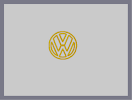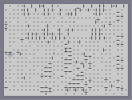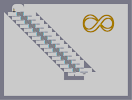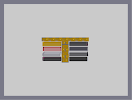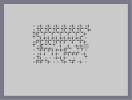Another line thing V W Another Frustarating Maze Infinity Myspace Buttons Another, yet less frustrating, maze.

Pages: (0)

### The title is Jag

So wouldn't you believe that it would turn into...a jaguar maybe?

### Ah,

I know what it is, now that I've seen the finished product.

### it ok

I was half way done and i wanted to see how it looked

### I think

its looking pretty cool. Hope u can make something really nice out of this...

### a swimmer?

...
That's half-way done? It looks more like 10% done to me. Oh well, I don't know what it's supposed to be anyway, so I can't say.
This is half way done.

You will know what it is when it is done.

### agrees with hoettii

What is it saposed to be?

### Its

looken cool but I don`t know what it could be...